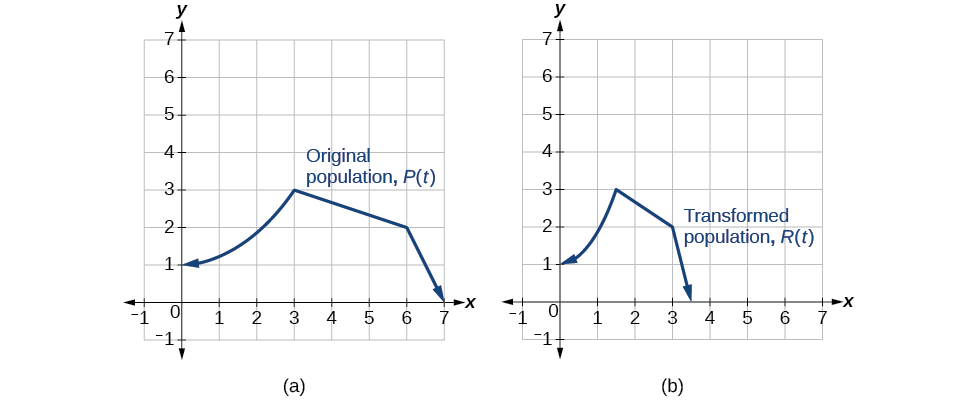# 3.5 Transformation of functions  (Page 8/21)

 Page 8 / 21

Write the formula for the function that we get when we stretch the identity toolkit function by a factor of 3, and then shift it down by 2 units.

$g\left(x\right)=3x-2$

## Horizontal stretches and compressions

Now we consider changes to the inside of a function. When we multiply a function’s input by a positive constant, we get a function whose graph is stretched or compressed horizontally in relation to the graph of the original function. If the constant is between 0 and 1, we get a horizontal stretch ; if the constant is greater than 1, we get a horizontal compression of the function.

Given a function $\text{\hspace{0.17em}}y=f\left(x\right),\text{\hspace{0.17em}}$ the form $\text{\hspace{0.17em}}y=f\left(bx\right)\text{\hspace{0.17em}}$ results in a horizontal stretch or compression. Consider the function $\text{\hspace{0.17em}}y={x}^{2}.\text{\hspace{0.17em}}$ Observe [link] . The graph of $\text{\hspace{0.17em}}y={\left(0.5x\right)}^{2}\text{\hspace{0.17em}}$ is a horizontal stretch of the graph of the function $\text{\hspace{0.17em}}y={x}^{2}\text{\hspace{0.17em}}$ by a factor of 2. The graph of $\text{\hspace{0.17em}}y={\left(2x\right)}^{2}\text{\hspace{0.17em}}$ is a horizontal compression of the graph of the function $\text{\hspace{0.17em}}y={x}^{2}\text{\hspace{0.17em}}$ by a factor of 2.

## Horizontal stretches and compressions

Given a function $\text{\hspace{0.17em}}f\left(x\right),\text{\hspace{0.17em}}$ a new function $\text{\hspace{0.17em}}g\left(x\right)=f\left(bx\right),\text{\hspace{0.17em}}$ where $\text{\hspace{0.17em}}b\text{\hspace{0.17em}}$ is a constant, is a horizontal stretch    or horizontal compression    of the function $\text{\hspace{0.17em}}f\left(x\right).$

• If $\text{\hspace{0.17em}}b>1,\text{\hspace{0.17em}}$ then the graph will be compressed by $\text{\hspace{0.17em}}\frac{1}{b}.$
• If $\text{\hspace{0.17em}}0 then the graph will be stretched by $\text{\hspace{0.17em}}\frac{1}{b}.$
• If $\text{\hspace{0.17em}}b<0,\text{\hspace{0.17em}}$ then there will be combination of a horizontal stretch or compression with a horizontal reflection.

Given a description of a function, sketch a horizontal compression or stretch.

1. Write a formula to represent the function.
2. Set $\text{\hspace{0.17em}}g\left(x\right)=f\left(bx\right)\text{\hspace{0.17em}}$ where $\text{\hspace{0.17em}}b>1\text{\hspace{0.17em}}$ for a compression or $\text{\hspace{0.17em}}0 for a stretch.

## Graphing a horizontal compression

Suppose a scientist is comparing a population of fruit flies to a population that progresses through its lifespan twice as fast as the original population. In other words, this new population, $\text{\hspace{0.17em}}R,\text{\hspace{0.17em}}$ will progress in 1 hour the same amount as the original population does in 2 hours, and in 2 hours, it will progress as much as the original population does in 4 hours. Sketch a graph of this population.

Symbolically, we could write

See [link] for a graphical comparison of the original population and the compressed population.(a) Original population graph (b) Compressed population graph

## Finding a horizontal stretch for a tabular function

A function $\text{\hspace{0.17em}}f\left(x\right)\text{\hspace{0.17em}}$ is given as [link] . Create a table for the function $\text{\hspace{0.17em}}g\left(x\right)=f\left(\frac{1}{2}x\right).$

 $x$ 2 4 6 8 $f\left(x\right)$ 1 3 7 11

The formula $\text{\hspace{0.17em}}g\left(x\right)=f\left(\frac{1}{2}x\right)\text{\hspace{0.17em}}$ tells us that the output values for $\text{\hspace{0.17em}}g\text{\hspace{0.17em}}$ are the same as the output values for the function $\text{\hspace{0.17em}}f\text{\hspace{0.17em}}$ at an input half the size. Notice that we do not have enough information to determine $\text{\hspace{0.17em}}g\left(2\right)\text{\hspace{0.17em}}$ because $\text{\hspace{0.17em}}g\left(2\right)=f\left(\frac{1}{2}\cdot 2\right)=f\left(1\right),\text{\hspace{0.17em}}$ and we do not have a value for $\text{\hspace{0.17em}}f\left(1\right)\text{\hspace{0.17em}}$ in our table. Our input values to $\text{\hspace{0.17em}}g\text{\hspace{0.17em}}$ will need to be twice as large to get inputs for $\text{\hspace{0.17em}}f\text{\hspace{0.17em}}$ that we can evaluate. For example, we can determine $\text{\hspace{0.17em}}g\left(4\right)\text{.}$

$g\left(4\right)=f\left(\frac{1}{2}\cdot 4\right)=f\left(2\right)=1$

We do the same for the other values to produce [link] .

 $x$ 4 8 12 16 $g\left(x\right)$ 1 3 7 11

[link] shows the graphs of both of these sets of points.

## Recognizing a horizontal compression on a graph

Relate the function $\text{\hspace{0.17em}}g\left(x\right)\text{\hspace{0.17em}}$ to $\text{\hspace{0.17em}}f\left(x\right)\text{\hspace{0.17em}}$ in [link] .

The graph of $\text{\hspace{0.17em}}g\left(x\right)\text{\hspace{0.17em}}$ looks like the graph of $\text{\hspace{0.17em}}f\left(x\right)\text{\hspace{0.17em}}$ horizontally compressed. Because $\text{\hspace{0.17em}}f\left(x\right)\text{\hspace{0.17em}}$ ends at $\text{\hspace{0.17em}}\left(6,4\right)\text{\hspace{0.17em}}$ and $\text{\hspace{0.17em}}g\left(x\right)\text{\hspace{0.17em}}$ ends at $\text{\hspace{0.17em}}\left(2,4\right),\text{\hspace{0.17em}}$ we can see that the $\text{\hspace{0.17em}}x\text{-}$ values have been compressed by $\text{\hspace{0.17em}}\frac{1}{3},\text{\hspace{0.17em}}$ because $\text{\hspace{0.17em}}6\left(\frac{1}{3}\right)=2.\text{\hspace{0.17em}}$ We might also notice that $\text{\hspace{0.17em}}g\left(2\right)=f\left(6\right)\text{\hspace{0.17em}}$ and $\text{\hspace{0.17em}}g\left(1\right)=f\left(3\right).\text{\hspace{0.17em}}$ Either way, we can describe this relationship as $\text{\hspace{0.17em}}g\left(x\right)=f\left(3x\right).\text{\hspace{0.17em}}$ This is a horizontal compression by $\text{\hspace{0.17em}}\frac{1}{3}.$

#### Questions & Answers

find to nearest one decimal place of centimeter the length of an arc of circle of radius length 12.5cm and subtending of centeral angle 1.6rad
factoring polynomial
find general solution of the Tanx=-1/root3,secx=2/root3
find general solution of the following equation
Nani
the value of 2 sin square 60 Cos 60
0.75
Lynne
0.75
Inkoom
when can I use sin, cos tan in a giving question
depending on the question
Nicholas
I am a carpenter and I have to cut and assemble a conventional roof line for a new home. The dimensions are: width 30'6" length 40'6". I want a 6 and 12 pitch. The roof is a full hip construction. Give me the L,W and height of rafters for the hip, hip jacks also the length of common jacks.
John
I want to learn the calculations
where can I get indices
I need matrices
Nasasira
hi
Raihany
Hi
Solomon
need help
Raihany
maybe provide us videos
Nasasira
Raihany
Hello
Cromwell
a
Amie
What do you mean by a
Cromwell
nothing. I accidentally press it
Amie
you guys know any app with matrices?
Khay
Ok
Cromwell
Solve the x? x=18+(24-3)=72
x-39=72 x=111
Suraj
Solve the formula for the indicated variable P=b+4a+2c, for b
Need help with this question please
b=-4ac-2c+P
Denisse
b=p-4a-2c
Suddhen
b= p - 4a - 2c
Snr
p=2(2a+C)+b
Suraj
b=p-2(2a+c)
Tapiwa
P=4a+b+2C
COLEMAN
b=P-4a-2c
COLEMAN
like Deadra, show me the step by step order of operation to alive for b
John
A laser rangefinder is locked on a comet approaching Earth. The distance g(x), in kilometers, of the comet after x days, for x in the interval 0 to 30 days, is given by g(x)=250,000csc(π30x). Graph g(x) on the interval [0, 35]. Evaluate g(5)  and interpret the information. What is the minimum distance between the comet and Earth? When does this occur? To which constant in the equation does this correspond? Find and discuss the meaning of any vertical asymptotes.
The sequence is {1,-1,1-1.....} has
circular region of radious
how can we solve this problem
Sin(A+B) = sinBcosA+cosBsinA
Prove it
Eseka
Eseka
hi
Joel
yah
immyByByByByBy Anonymous UserBy Lakeima RobertsBy